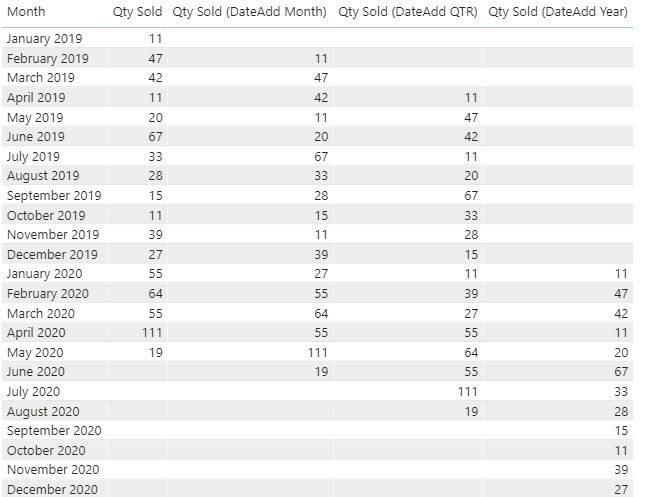top of page
Search

# DAX Time Intelligence Part 1: IntroductionThe date is an essential aspect of a data model. An analyst requires comparing the current period’s performance with multiple reference points in history. For example, analyzing the performance for May against:

· Last month (April)

· May of last year

· MTD, QTD, & YTD aggregations for current and previous years

DAX offers Time Intelligence functions in this context, which makes the job easier. This article covers some of the essential Time Intelligence functions: PREVIOUSMONTH, SAMEPERIODLASTYEAR, DATEADD, PARALLELPERIOD, PREVIOUSQUARTER, PREVIOUSYEAR

## Sample Data

Let us consider a simple sample data table for this exercise:It has data from Jan 2019 to May 2020.

Ideally, a data model should contain a date/calendar table. By using a calendar table, it is easier to browse the model, and we can use specific DAX functions that perform time intelligence calculations. As a fact, most of the time intelligence functions in DAX require a separate date table.

For this exercise, we have added a simple calendar table:

(Modelling > New Table, and then insert the following code)

```ftCalendar =
CALENDARAUTO(),
"Year", YEAR([Date]),
"Month", EOMONTH([Date],-1)+1,
"MonthNum",MONTH([Date]),
"MonthName", Format([Date],"mmm"),
"Qtr", "Q" & FORMAT([Date],"q"),
"YearQtr", YEAR([Date]) & " Q" & FORMAT([Date],"q"),

//Calculating Financial year ending on March 31
"FY Year", IF(MONTH([Date])>3,"FY "&YEAR([Date])&"-
"&RIGHT(YEAR([Date])+1,2),"FY "&YEAR([Date])-1&"-
"&RIGHT(YEAR([Date]),2)),

//Calculating Financial Qtr
"FY Qtr", "Q"&CEILING(MONTH(EOMONTH([Date],-3)),3)/3
)```

Establish a relationship between the data table and the calendar table.

Note: Once created, all date references in the Time Intelligence DAX shall be from the calendar table.

The following measures also have been added in the model:

``` Qty Sold = SUM(dtTable[Qty])

Sales Amount =
SUMX(
dtTable,
dtTable[Price Per Unit] * dtTable[Qty]
)

Cost Amount =
SUMX(
dtTable,
dtTable[Cost Per Unit] * dtTable[Qty]
)

Profit = [Sales Amount] - [Cost Amount]
```

A monthly summary of the data table on all the measures:## Calculating Values for Previous Month

To calculate the values of the previous month, let us say for Qty Sold, we write the following set of codes:

``` Qty Sold Prev Month =
VAR _CurrentDate = MAX(ftCalendar[Date])
VAR _StartDate = EOMONTH(_CurrentDate,-2)+1
VAR _EndDate = EOMONTH(_StartDate,0)

//Filter the date table for the above range
VAR _Filter =
FILTER(
ALL(ftCalendar[Date]),
ftCalendar[Date] >= _StartDate
&& ftCalendar[Date] <= _EndDate
)

//Calculating Qty Sold for the filtered range
VAR _PrevMonth_QtySold =
CALCULATE(
[Qty Sold],
_Filter
)

RETURN
_PrevMonth_QtySold
```

## Using PREVIOUSMONTH

Alternatively, we can take the help of PREVIOUSMONTH and simplify the code:

``` Qty Sold (Prev Month) =
CALCULATE(
[Qty Sold],
PREVIOUSMONTH(ftCalendar[Date])
)
```In Essence, time intelligence functions are doing the same thing that we are achieving through writing FILTER functions. The only difference is that time intelligence functions are simpler to write. And since the names of the time intelligence functions are quite intuitive, it makes our lives easier. Let us consider another example

## Using SAMEPERIODLASTYEAR

As the name suggests, it shifts the set of dates by one year:

``` Qty Sold (SPLY) =
CALCULATE(
[Qty Sold],
SAMEPERIODLASTYEAR(ftCalendar[Date])
)
```

Against January 2020, it shows values for Jan 2019.SAMEPERIODLASTYEAR is a specific version of DATEADD, where it shifts only the year. DATEADD function provides more options in this regard. It takes the number and type of period to shift, which includes YEAR, QUARTER, MONTH, and DAY.

The following formulas showing

``` Qty Sold (DateAdd Month) =
CALCULATE(
[Qty Sold],
)

CALCULATE(
[Qty Sold],
)

CALCULATE(
[Qty Sold],
)
```## Using PARALLELPERIOD

PARALLELPERIOD is similar to DATEADD, but it returns the full period specified in the parameter. In other words, PARALLELPERIOD returns a range of dates.

PARALLELPERIOD requires three arguments:In this case, to calculate Qty Sold for the previous month, we use the following inputs for the arguments:

``` Qty Sold (PP Month) =
CALCULATE(
[Qty Sold],
PARALLELPERIOD(ftCalendar[Date],-1,MONTH)
)

Qty Sold (PP QTR) =
CALCULATE(
[Qty Sold],
PARALLELPERIOD(ftCalendar[Date],-1,QUARTER)
)

Qty Sold (PP Year) =
CALCULATE(
[Qty Sold],
PARALLELPERIOD(ftCalendar[Date],-1,YEAR)
)
```Essentially, we are telling DAX to:

• Group the calendar table date field on month, quarter, or year

• Shift to one period before the current month value

• filter the calendar table for dates falling in the period

## Using PREVIOUSQUARTER & PREVIOUSYEAR

Similar to PREVIOUSMONTH, we have DAX which returns a full period for the specified duration:

``` Qty Sold (Prev Qtr) =
CALCULATE(
[Qty Sold],
PREVIOUSQUARTER(ftCalendar[Date])
)

Qty Sold (Prev Year) =
CALCULATE(
[Qty Sold],
PREVIOUSYEAR(ftCalendar[Date])
)
```## Difference between PARALLELPERIOD and PREVIOUSMONTH/QUARTER/YEAR

If multiple periods are selected, then PARALLELPERIOD returns a shifted result.

PREVIOUS(year, quarter, month, or day) always returns a single element adjoining to the selected period.We can use other measures instead of Qty Sold to compare and analyze performance.

``` Profit (Prev Month) =
CALCULATE(
[Profit],
PREVIOUSMONTH(ftCalendar[Date])
)

Profit (Prev QTR) =
CALCULATE(
[Profit],
PREVIOUSQUARTER(ftCalendar[Date])
)

Profit (Prev Year) =
CALCULATE(
[Profit],
PREVIOUSYEAR(ftCalendar[Date])
)
```Check the article on aggregations with Time Intelligence

Time Intelligence part 2: Aggregations

@imVivRan

bottom of page